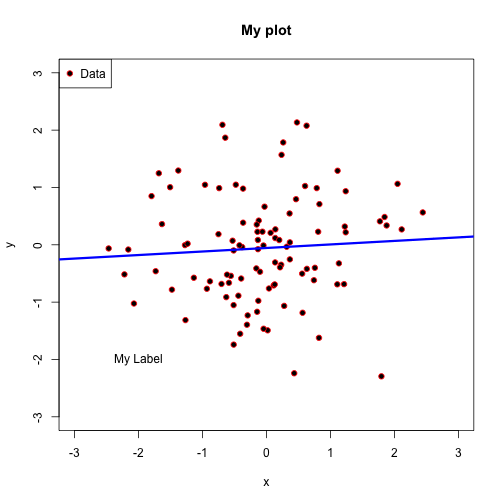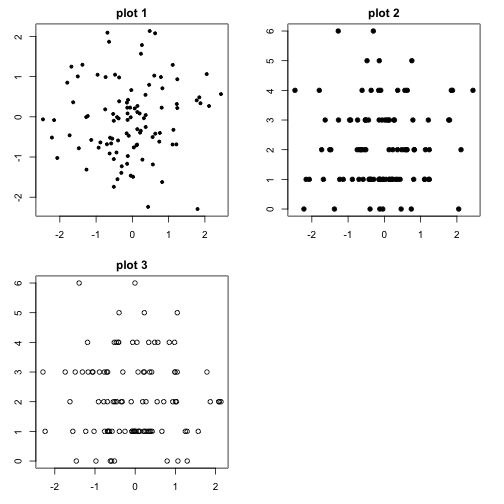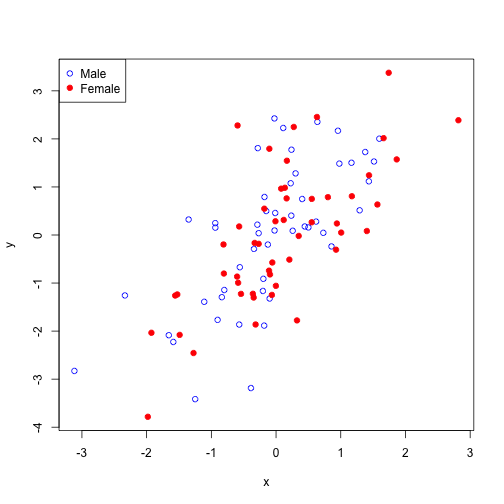Important parameters

• pch: plotting symbol
• lty: line type
• lwd: line width
• col: plotting color
• las: orientation of axis labels
• bg: background color
• mar: margin size
• oma: outer margin size
• mfrow: number of plots per row, column. Plots filled in row-wise.
• mfcol: number of plots per row, column. Plots filled in column-wise.

Import functions

• plot: make scatterplot
• lines: add lines to plot
• points: add points to plot
• title: add title to axes or plot

Example 1

x <- rnorm(100)
y <- rnorm(100)
plot(x, y, pch = 21, mar = c(4, 4, 2, 2), col = "red", bg = "black", xlim = c(-3,
3), ylim = c(-3, 3))
fit <- lm(y ~ x)
abline(fit, lwd = 3, col = "blue")
title("My plot")
text(-2, -2, "My Label")
legend("topleft", legend = "Data", pch = 21, pt.bg = "black", col = "red")Example 2

To use multiple plots per page, give a mfrow argument to plot. Then the plots will be filled from left to right across the rows. (Specifying mfcol will fill in plots from top to bottom down the columns. )

z <- rpois(100, 2)
plot.new()
par(mfrow = c(2, 2))
par(mar = c(3, 3, 2, 2))
plot(x, y, pch = 20, main = "plot 1")
plot(x, z, pch = 19, main = "plot 2")
plot(y, z, pch = 1, main = "plot 3")Example 3

The points function is useful for adding points to a plot for different groups.

plot.new()
x <- rnorm(100)
y <- x + rnorm(100)
g <- gl(2, 50, labels = c("Male", "Female"))
str(g)
##  Factor w/ 2 levels "Male","Female": 1 1 1 1 1 1 1 1 1 1 ...
plot(x, y, type = "n")  # Draws no points
points(x[g == "Male"], y[g == "Male"], col = "blue", pch = 1)
points(x[g == "Female"], y[g == "Female"], col = "red", pch = 19)
legend("topleft", c("Male", "Female"), col = c("blue", "red"), pch = c(1, 19))Go Top